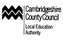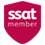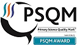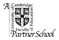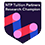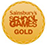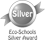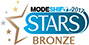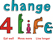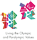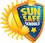# Learning Expectations in Year 5

End of Year 5 Learning Expectations

Word reading

• I can apply knowledge of root words, prefixes and suffixes to read aloud and to understand the meaning of unfamiliar words.
• I can read further exception words, noting the unusual correspondences between spelling and sound.
• I attempt pronunciation of unfamiliar words drawing on prior knowledge of similar looking words.
• I can re-read and read ahead to check for meaning.

Understanding what I read

• I am familiar with and can talk about a wide range of books and text types, including myths, legends and traditional stories and books from other cultures and traditions. I can discuss the features of each.
• I can read non-fiction texts and identify the purpose, structure and grammatical features, evaluating how effective they are.
• I can identify significant ideas, events and characters; and discuss their significance.
• I can recite poems by heart, e.g. narrative verse, haiku.
• I can prepare poems and plays to read aloud and to perform, showing understanding through intonation, tone, volume and action.

Spelling

• I can form verbs with prefixes.
• I can convert nouns or adjectives into verbs by adding a suffix.
• I understand the rules for adding prefixes and suffixes.
• I can spell words with silent letters.
• I can distinguish between homophones and other words which are often confused.
• I can spell the commonly mis-spelt words from the Y5/6 word list.
• I can use the first 3 or 4 letters of a word to check spelling, meaning or both in a dictionary.
• I can use a thesaurus.
• I can use a range of spelling strategies.

Handwriting

• I can choose the style of handwriting to use when given a choice.
• I can choose the handwriting that is best suited for a specific task.

Sentence structure

• I can use relative clauses.
• I can use adverbs or modal verbs to indicate a degree of possibility.

Text structure

• I can build cohesion between paragraphs.
• I can use adverbials to link paragraphs.

Punctuation

• I can use inverted commas and other punctuation to indicate direct speech.
• I can use apostrophes to mark plural possession.
• I use commas after fronted adverbials.
• I can use brackets, dashes and commas to indicate parenthesis.
• I can use commas to clarify meaning or avoid ambiguity.

Number

• I can count forwards and backwards in steps of powers of 10 for any given number up to 1,000,000.
• I recognise mixed numbers and improper fractions and can convert from one to the other.
• I can read and write decimal numbers as fractions.
• I recognise the % symbol and understand percent relates to a number of parts per hundred.
• I can write percentages as a fraction with denominator hundred and as a decimal fraction.
• I can compare and add fractions whose denominators are all multiples of the same number.
• I can multiply and divide numbers mentally drawing on known facts up to 12 x
• I can round decimals with 2dp to the nearest whole number and to 1dp.
• I recognise and use square numbers and cube numbers; and can use the notation and 3 .
• I can multiply and divide whole numbers and those involving decimals by 10, 100 and 1000.
• I can multiply numbers up to 4-digit by a 1 or 2-digit number using formal written methods, including long multiplication for a 2-digit number.
• I can divide numbers up to 4-digits by a 1-digit number.
• I can solve problems involving multiplication and division where large numbers are used by decomposing them into factors.
• I can solve addition and subtraction multi-step problems in context, deciding which operations and methods to use and why.
• I can solve problems involving numbers up to 3dp.

Measurement, geometry and statistics

• I can estimate and compare acute, obtuse and reflex angles.
• I can draw given angles and measure them in degrees.
• I can convert between different units of metric measures and estimate volume and capacity.
•  I can measure and calculate the perimeter of composite rectilinear shapes in cm and m.
•  I can calculate and compare the areas of squares and rectangles including using standards units (cm2 and m2 ).
• I can solve comparison, sum and difference problems using information presented in a line graph.